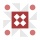Home
IT Knowledge
Inspiration
Languages
EN

# Python - get dictionary values

0 points
Created by:Niac
208

In this article, we would like to show you how to get dictionary values in Python.

Quick solution:

``my_dictionary.values()``

## Practical example

In this example, we use `values()` method to get the list of `my_dict` dictionary values.

``````my_dict = {
"id": 1,
"name": "Tom",
"age": 25
}
result = my_dict.values()

print(result)  # dict_values([1, 'Tom', 25])``````

Output:

``dict_values([1, 'Tom', 25])``